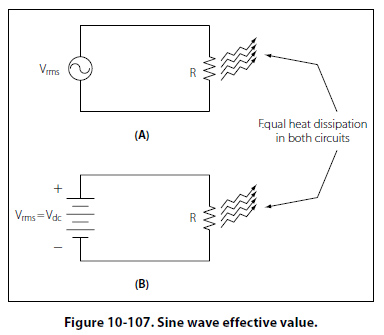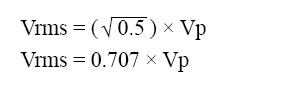Values of Alternating Current There are three values of alternating current, which are instantaneous, peak, and effective (root mean square, RMS). Instantaneous Value An instantaneous value of voltage or current is the induced voltage or current flowing at any instant during a cycle. The sine wave represents a series of these values. The instantaneous value of the voltage varies from zero at 0° to maximum at 90°, back to zero at 180°, to maximum in the opposite direction at 270°, and to zero again at 360°. Any point on the sine wave is considered the instantaneous value of voltage. Peak Value The peak value is the largest instantaneous value. The largest single positive value occurs when the sine wave of voltage is at 90°, and the largest single negative value occurs when it is at 270°. Maximum value is 1.41 times the effective value. These are called peak values. Effective Value The effective value is also known as the RMS value or root mean square, which refers to the mathematical process by which the value is derived. Most AC voltmeters will display the effective or RMS value when used. The effective value is less than the maximum value, being equal to .707 times the maximum value. The effective value of a sine wave is actually a measure of the heating effect of the sine wave. Figure 10-107 illustrates what happens when a resistor is connected across an AC voltage source. In illustration A, a certain amount of heat is generated by the power in the resistor. Illustration B shows the same resistor now inserted into a DC voltage source. The value of the DC voltage source can now be adjusted so that the resistor dissipates the same amount of heat as it did when it was in the AC circuit. The RMS or effective value of a sine wave is equal to the DC voltage that produces the same amount of heat as the sinusoidal voltage.The peak value of a sine wave can be converted to the corresponding RMS value using the following relationship.This can be applied to either voltage or current. Algebraically rearranging the formula and solving for Vp can also determine the peak voltage. The resulting formula is: Vp = 1.414 × Vrms Thus, the 110 volt value given for alternating current supplied to homes is only 0.707 of the maximum voltage of this supply. The maximum voltage is approximately 155 volts (110 × 1.41 = 155 volts maximum). In the study of alternating current, any values given for current or voltage are assumed to be effective values unless otherwise specified, and in practice, only the effective values of voltage and current are used. Similarly, alternating current voltmeters and ammeters measure the effective value.
 ©AvStop Online Magazine                                                                                                                                                      Contact Us              Return To Books